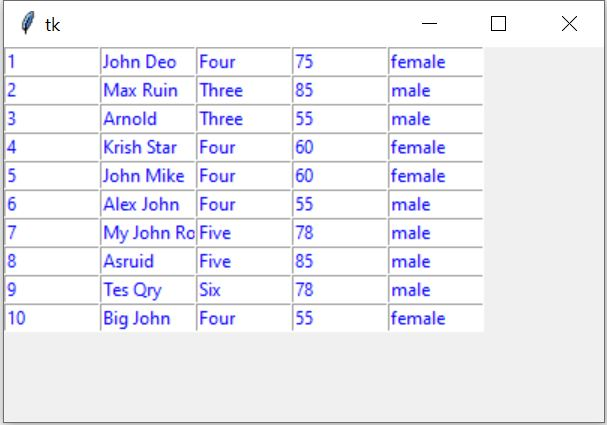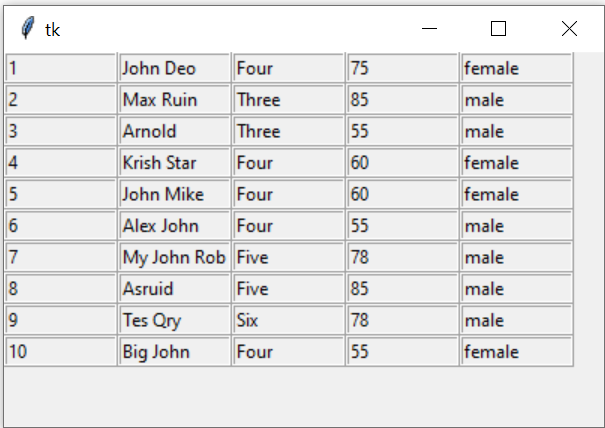# Displaying records from MySQL table

Display records from MySQL Student table in Tkinter window.Display ten records of student table of MySQL database on Tkinter window using SELECT & LIMIT Query

## Connect to MySQL database

We connected to MySQL database by using our userid, password and database name. You can update your MySQL login details here.
``````import mysql.connector
my_connect = mysql.connector.connect(
host="localhost",
user="userid",
database="database_name"
)
####### end of connection ####
my_conn = my_connect.cursor()``````
We will use my_conn in our further script as the connection object to get our records.

We will use Query with LIMIT to collect 10 records from the student table.

By using LIMIT Query we are restricting our number of records by getting 10 records only from MySQL database. You can change this figure to get different number of records from the database.

Note that MySQL database executes the query part only and return us one result set. This result set contains all our (10) records.
We used the returned MySQLCursor i.e my_conn as an iterator

We will use one for loop to collect each row of record from the data set. Each row of data ( here student ) is a tuple. So we used another for loop to display each element of this tuple.

While printing the elements of student ( a tuple ) no line break is added and once the printing of one row of data is completed ( without any line break ) then outside the for loop one line break is added by using print()
``````my_conn = my_connect.cursor()
my_conn.execute("SELECT * FROM student limit 0,10")
i=0
for student in my_conn:
for j in range(len(student)):
print(student[j],end='')
i=i+1
print()# line break at the end of one row``````
This will print 10 rows of records from student table.

## Using tkinter

We will use one tkinter entry component to display each data in the window. In this variable student is a tuple and it contains one row of data. We used variable i as index for each row and variable j as each column of data.
The full code is here , Change the userid,password and database name of your MySQL database.
``````import mysql.connector
import tkinter  as tk
from tkinter import *
my_w = tk.Tk()
my_w.geometry("400x250")
my_connect = mysql.connector.connect(
host="localhost",
user="userid",
database="database_name"
)

my_conn = my_connect.cursor()
####### end of connection ####
my_conn.execute("SELECT * FROM student limit 0,10")
i=0
for student in my_conn:
for j in range(len(student)):
e = Entry(my_w, width=10, fg='blue')
e.grid(row=i, column=j)
e.insert(END, student[j])
i=i+1
my_w.mainloop()``````

## Using SQLAlchemy

We can use SQLAlchemy library to manage MySQL database. Here is the same code using SQLAlchemy. Connection part is highlighted. Here also you have to change your MySQL login details like userid, password and database name.
``````import tkinter  as tk
from tkinter import *
my_w = tk.Tk()
my_w.geometry("400x250")
from sqlalchemy import create_engine
####### end of connection ####
r_set=my_conn.execute("SELECT * FROM student limit 0,10")
i=0
for student in r_set:
for j in range(len(student)):
e = Entry(my_w, width=10, fg='blue')
e.grid(row=i, column=j)
e.insert(END, student[j])
i=i+1
my_w.mainloop()``````
Using Label in place of Entry in above code.
``````for student in r_set:
for j in range(len(student)):
e = Label(my_w,width=10, text=student[j])
e.grid(row=i, column=j)
#e.insert(END, student[j])
i=i+1``````
We can add border to each cell by using `borderwidth=2`, `relief='ridge'` and align the text to left by using `anchor='w'`.
``````e = Label(my_w,width=10, text=student[j],
borderwidth=2,relief='ridge', anchor="w") ``````Displaying MySQL records & adding border with alignment and showing column names as header

Note that if column header row is starting from first row ( row=0 ) then i value has to start from 1. We are using Labels to add one row with five columns. Use this code before the for loop.``````e=Label(my_w,width=10,text='id',borderwidth=2, relief='ridge',anchor='w',bg='yellow')
e.grid(row=0,column=0)
e=Label(my_w,width=10,text='Name',borderwidth=2, relief='ridge',anchor='w',bg='yellow')
e.grid(row=0,column=1)
e=Label(my_w,width=10,text='Class',borderwidth=2, relief='ridge',anchor='w',bg='yellow')
e.grid(row=0,column=2)
e=Label(my_w,width=10,text='mark',borderwidth=2, relief='ridge',anchor='w',bg='yellow')
e.grid(row=0,column=3)
e=Label(my_w,width=10,text='Gender',borderwidth=2, relief='ridge',anchor='w',bg='yellow')
e.grid(row=0,column=4)
i=1``````

## Pagination of records

If you have more records to display then you can break the total records in parts and display fixed number of records.

Subscribe to our YouTube Channel here

## Subscribe

* indicates required
Subscribe to plus2netplus2net.com

 01-11-2020 Thanks a lot...Loved it!! It works for me!
 21-11-2020 Thanks a lot
 02-12-2020 Hey, your code is working very well. Thank You so much. But there is one query. Every time the table is displayed in the Tk() window it goes to the top left corner. What is to be done if i want it somewhere in the middle?
 04-12-2020 my_w.geometry('400x250+450+350') Here 450 and 350 are x and y position. You can change this value to position it at different locations. You can further improve it by reading user screen resolution and then apply it to dynamically position the window by changing these values.
 04-12-2020 Don't use space while adding values to geometry(). This will not work my_w.geometry('400x250 + 450 + 350')
 02-07-2021 thank you soo much !!!!!!!!!!!!
 20-07-2023 Hi, this is working very well for me, how do I make the labels update each time I make a change to the database? thank you
 22-07-2023 You have to refresh the view to reflect the changes. Here we are using grid view so there is a way to refresh the grid view. Grid view details are here, Grid use grid_forget() to remove than and create again.